### Home > PC > Chapter 2 > Lesson 2.3.5 > Problem2-131

2-131.
1. For ΔABC below, state the ratio in terms of the sides. Homework Help ✎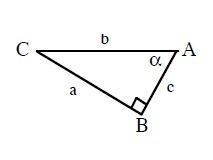1.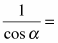?

2.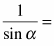?

3.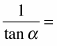?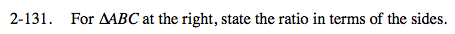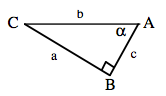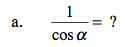$\frac{1}{\cos\alpha}=\frac{1}{c/b}$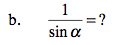sin(α) = a/b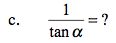tan(α) = a/c